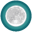FANDOM

1,895 Pages

Artisan ArithmeticianDescription
Achieve mastery in Multiplying decimals, Dividing decimals, Multiplying fractions, Dividing fractions
Details
TypeEnergy point(s) awarded 750
Status Retired
Disclosed?
Repeating?

Artisan Arithmetician is a Moon badge. To earn it, the user had to achieve mastery in several certain exercises:

• Multiplying decimals
• Dividing decimals
• Multiplying fractions
• Dividing fractions

This badge motivated users to become proficient by practicing more exercises, especially Arithmetic ones.